Private GMAT Tutoring in NYC & Online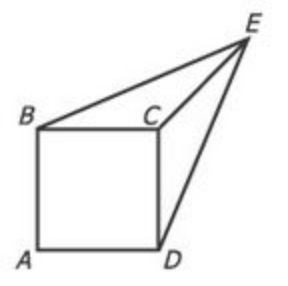In the figure above, each side of square ABCD has length 1, the length of line segment CE is 1, and the length of line segment BE is equal to the length of line segment DE. What is the area of the triangular region BCE?

(A) 1/3
(B) √2/4
(C) 1/2
(D) √2/2
(E) 3/4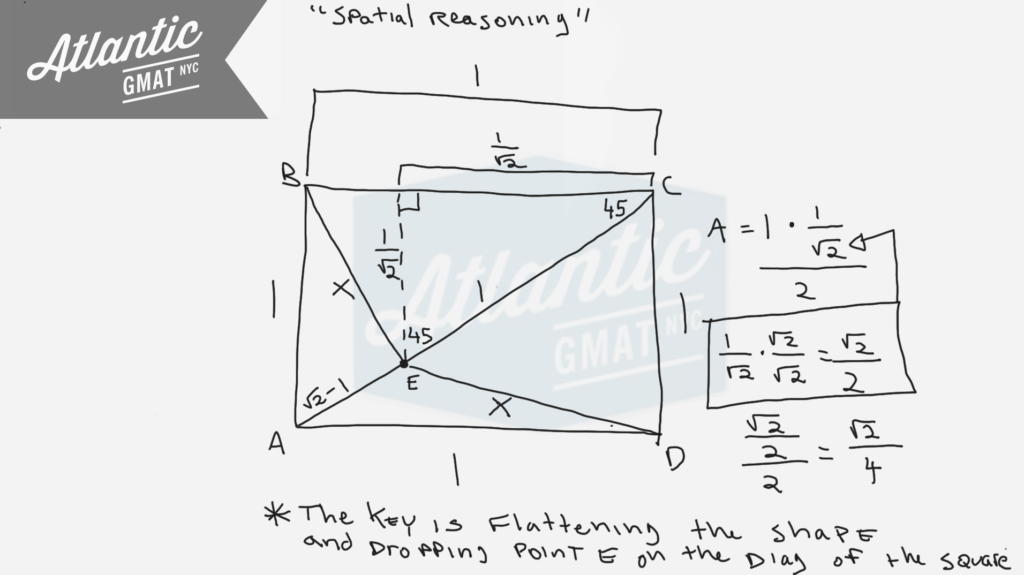A small, rectangular park has a perimeter of 560 feet and a diagonal measurement of 200 feet. What is its area, in square feet?

(A) 19,200
(B) 19,600
(C) 20,000
(D) 20,400
(E) 20,800

This is a very basic GMAT geometry question: area of a rectangle given the perimeter and diagonal. System of equations. Again, the setup is easy peasy. It gets a bit tricky once you need to simplify to an answer. Depending on how you approach the execution you might get stuck. In addition, the answer choices are bunched closely together so you can't really estimate/approximate.

What should you do if encountered with this type of situation on your GMAT? Panic! Just kidding. Remind yourself that there's likely a simple way to approach things. Remember that unless a number is prime you can pull it apart. Often then there's an easier "shape" to a number depending on what you're trying to do.

In this case, by pulling out some factors we can:

1. Do easier math with known multiples
2. Divide by two

Also, with exponents often you end up factoring. So given that you have a bunch of exponents present factoring should come to mind. These simple setup/tricky execution questions aren't uncommon so it's important to work on being flexible in how you approach your GMAT arithmetic. Hope this is helpful. Comment with any questions!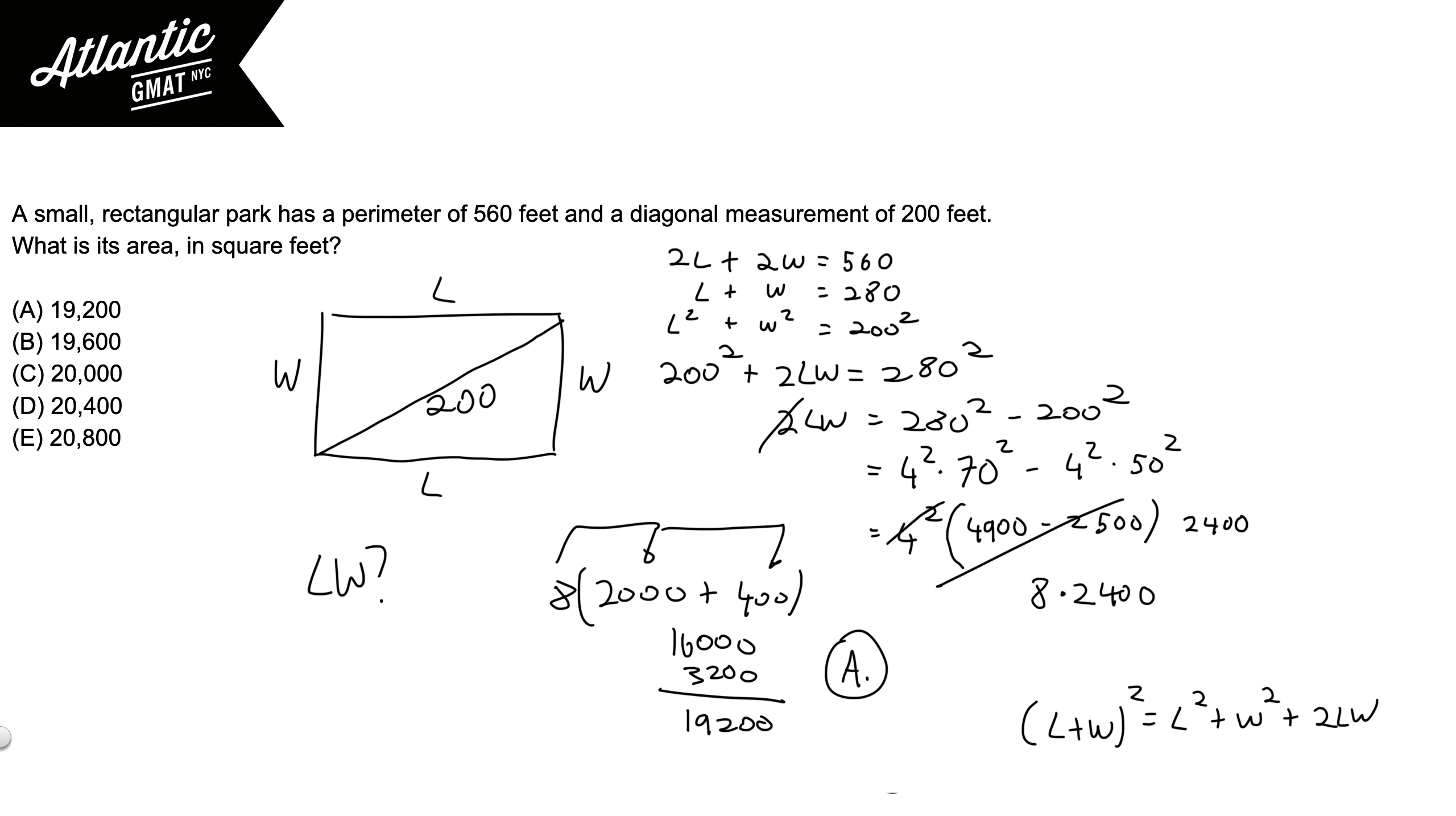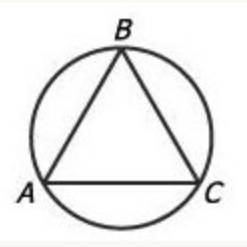In the figure above, equilateral triangle ABC is inscribed in the circle. If the length of arc ABC is 24, what is the approximate diameter of the circle?

(A) 5
(B) 8
(C) 11
(D) 15
(E) 19

It's key to know that an equilateral triangle has equal sides and equal angles measuring 60 degrees each. So each angle of an equilateral triangle inscribed in a circle cuts off 1/3 of the circle. Why is that? Review the basics! An angle on the circumference of a circle cuts off twice that angle's measure. So a 60 degree angle on the edge of a circle cuts off 120 degrees or 1/3 of a circle (120/360 degrees). A 60 degree angle in the center of a circle only cuts off 60 degrees (it doesn't have the extra space to open up). Examples below to illustrate this. Also, there's a special case for triangles that have the diameter of a circle as their base. Because the diameter is a 180 degree central angle, the angle opposite on the circumference of the circle must be 90 degrees. Not relevant for this question but good to know.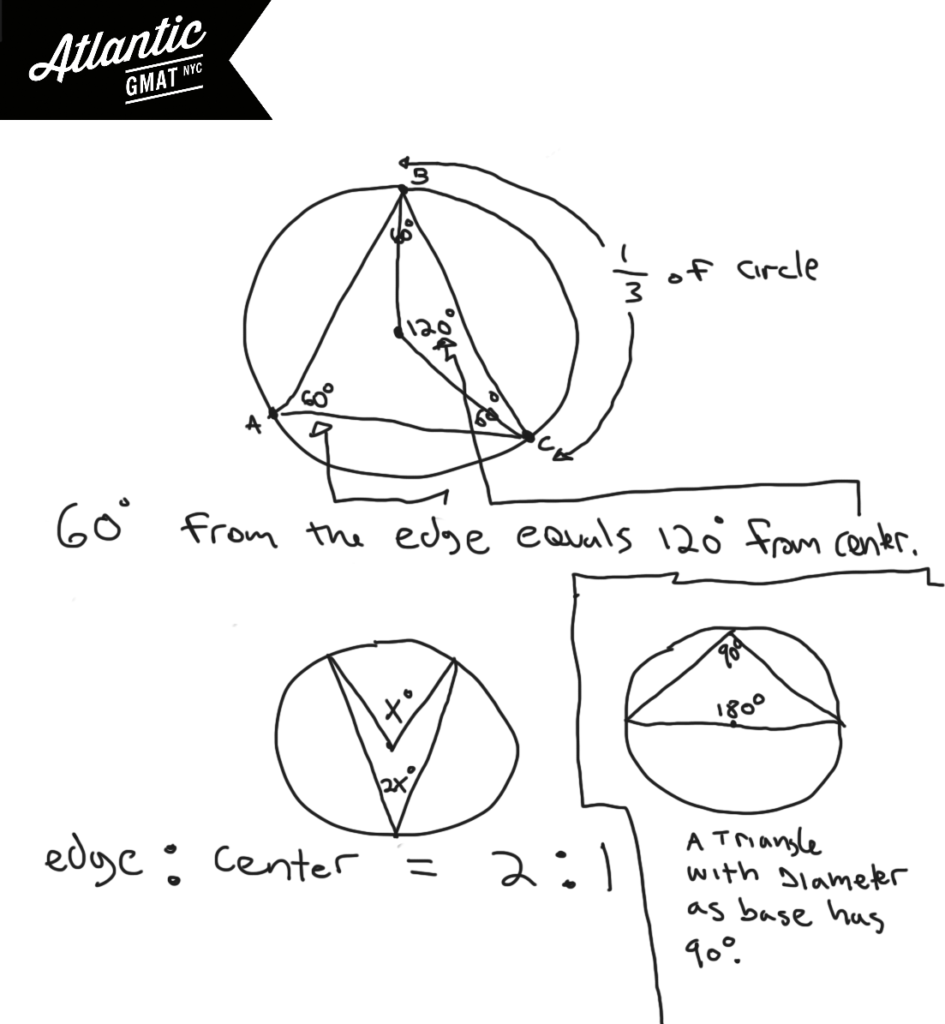How much of the circle is arc ABC? It's covering the same part of the circumference as two of the 60 degree angles from triangle ABA. Each of those angles represent 1/3 of the circle so arc ABC is 2/3 of the circle. If 2/3 is 24, 1/3 of the circle's circumference is 12, and the entire circumference is 36. So Pi*diameter = circumference. Pi*D = 36. D = 36/Pi. D = 36/3.14. A little less than 12. 11 is by far the closest answer choice. A little rusty feeling on these GMAT geometry rules? The rules are the easy part of GMAT studying. Get your fundamentals in shape ASAP. Avoid churning through questions before getting your basics GMAT quant organized. You don't want to waste good material and it's much harder to focus on the basics while working on tougher/trickier GMAT questions. Comment with any questions or additions. Happy studies!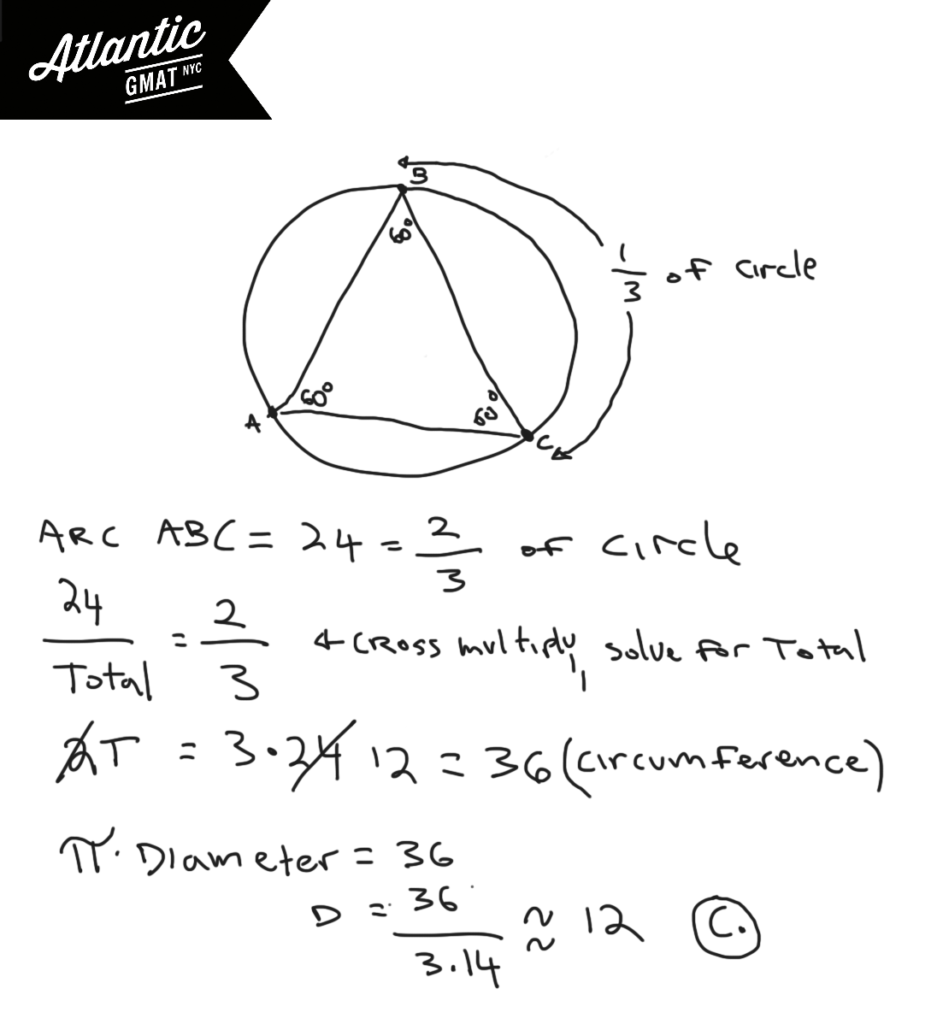A right circular cone is inscribed in a hemisphere so that the base of the cone coincides with the base of the hemisphere. What is the ratio of the height of the cone to the radius of the hemisphere?

(A) √3:1
(B) 1:1
(C) 1/2/1
(D) √2:1
(E) 2:1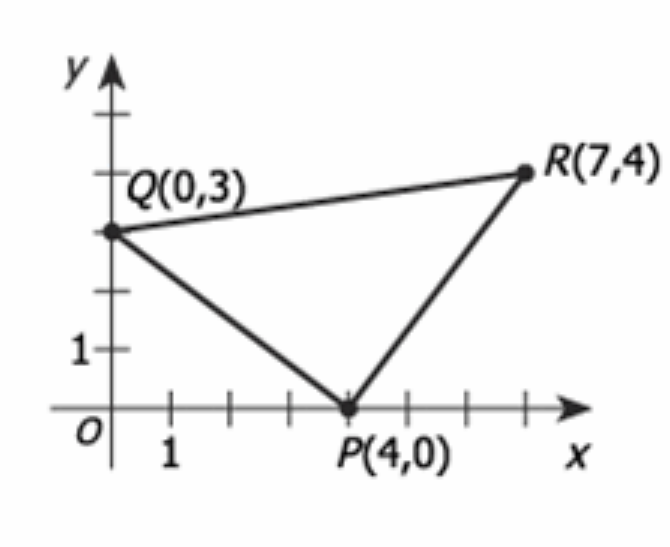In the rectangular coordinate system above, the area of triangular region PQR is

(A) 12.5

(B) 14

(C) 10√2

(D) 16

(E) 25

For GMAT geometry questions be active with diagrams. Don't be satisfied with what you're given. Try to make simple additions that provide more information (inferences). In general when trying to find an area on the coordinate plane you need to make a square, rectangle, or at the least, a right triangle. Right angles make measurement possible. Also, be open to the idea of adding/subtracting the areas of two or more shapes in order to solve for the desired area. In this case I'd draw two extra lines to make a rectangle.With that you should notice that you've created extra triangles and that those are right triangles. On GMAT geometry right triangles are your friends because you can do Pythagorean theorem and calculating are uses on the sides of the triangle (there's no need to drop an altitude). Now you can calculate the area of the rectangle and then subtract from that the areas of the three right triangles. That will leave the area of the middle triangle. Comment with any question or additions. Happy studies!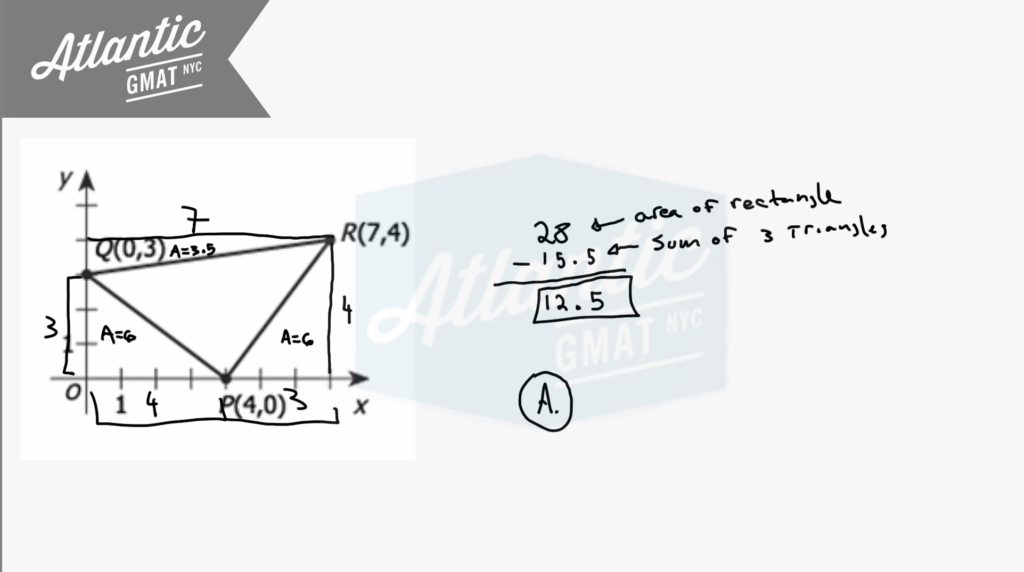To see it done live here is a video solution:

CONTACT

Atlantic GMAT

405 East 51st St.

NY, NY 10022

(347) 669-3545

info@AtlanticGMAT.com